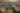# Learning Invariances using the Marginal Likelihood

Date December 2, 2018
Authors Mark van der Wilk , Matthias Bauer (University of Cambridge and the Max Planck institute in Tübingen), ST John , James Hensman

Generalising well in supervised learning tasks relies on correctly extrapolating the training data to a large region of the input space. One way to achieve this is to constrain the predictions to be invariant to transformations on the input that are known to be irrelevant (e.g. translation). Commonly, this is done through data augmentation, where the training set is enlarged by applying hand-crafted transformations to the inputs. We argue that invariances should instead be incorporated in the model structure, and learned using the marginal likelihood, which correctly rewards the reduced complexity of invariant models. We demonstrate this for Gaussian process models, due to the ease with which their marginal likelihood can be estimated. Our main contribution is a variational inference scheme for Gaussian processes containing invariances described by a sampling procedure. We learn the sampling procedure by back-propagating through it to maximise the marginal likelihood.

View the paper

Share
,,